# A 3-turn coil encloses an elliptical area having a major axis of L = 42.0 cm...

A 3-turn coil encloses an elliptical area having a major axis of L = 42.0 cm and a minor axis of W = 28.9 cm, as seen in the figure. The coil lies in the plane of the page and has an I = 5.65 A current flowing clockwise in it. If the coil is in a uniform magnetic field of B = 1.62×10-4 T, directed toward the left of the page, what is the magnitude of the torque on the coil?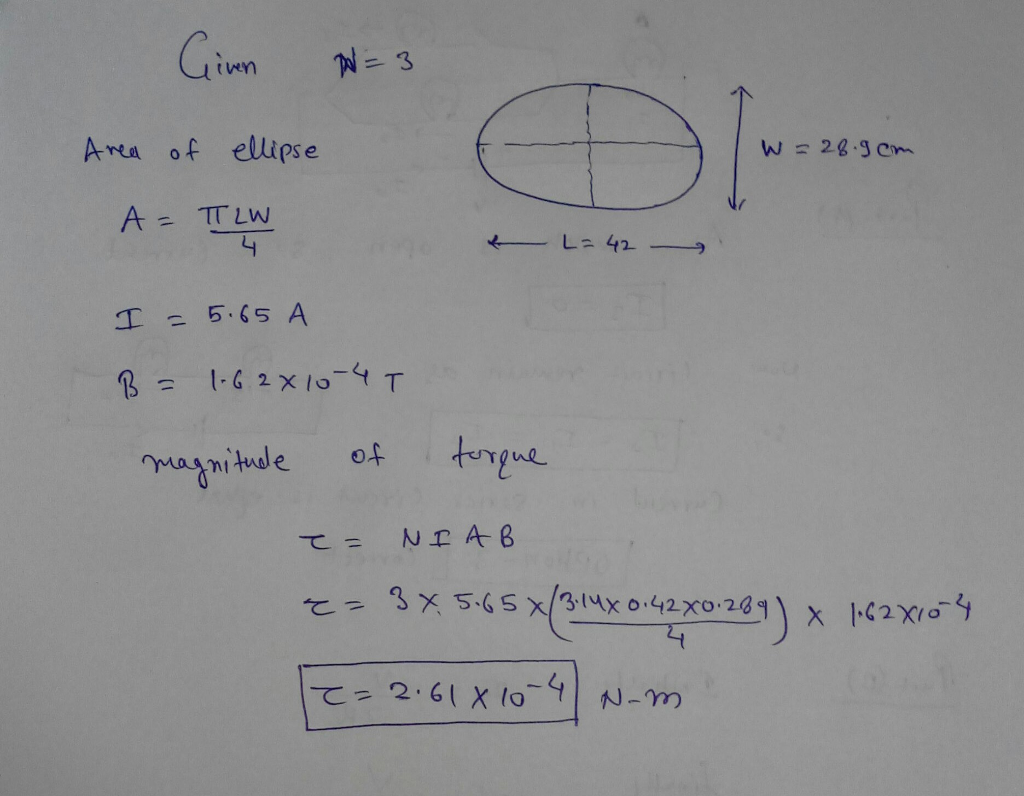##### Add Answer to: A 3-turn coil encloses an elliptical area having a major axis of L = 42.0 cm...
Similar Homework Help Questions
• ### K W A 5-turn coil encloses an elliptical area having a major axis of L =...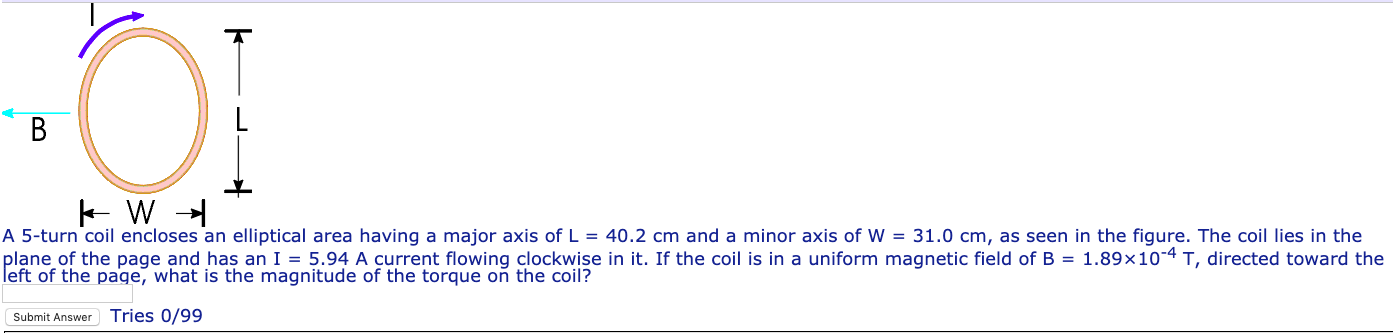K W A 5-turn coil encloses an elliptical area having a major axis of L = 40.2 cm and a minor axis of W = 31.0 cm, as seen in the figure. The coil lies in the plane of the page and has an I = 5.94 A current flowing clockwise in it. If the coil is in a uniform magnetic field of B = 1.89x10-4 T, directed toward the left of the page, what is the magnitude of the...

• ### An eight-turn coil encloses an elliptical area having a major axis of 40.0 cm and a...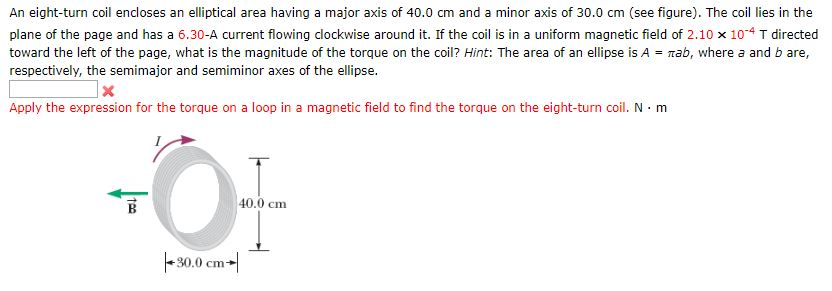An eight-turn coil encloses an elliptical area having a major axis of 40.0 cm and a minor axis of 30.0 cm (see figure). The coil lies in the plane of the page and has a 6.30-A current flowing clockwise around it. If the coil is in a uniform magnetic field of 2.10 x 104 T directed toward the left of the page, what is the magnitude of the torque on the coil? Hint: The area of an ellipse is A...

• ### An eight-turn coil encloses an elliptical area having a major axis of 40.0 cm and a...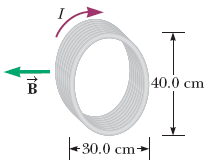An eight-turn coil encloses an elliptical area having a major axis of 40.0 cm and a minor axis of 30.0 cm (see figure). The coil lies in the plane of the page and carries a clockwise current of 6.40 A. If the coil is in a uniform magnetic field of 1.96 10-4 T directed toward the left of the page, what is the magnitude of the torque on the coil? Hint: The area of an ellipse is A = πab,...

• ### An eight-turn coil encloses an elliptical area having a major axis of 40.0 cm and a...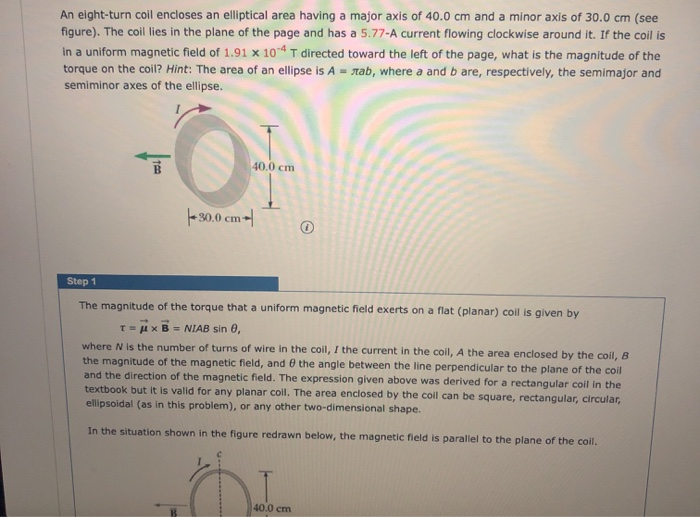An eight-turn coil encloses an elliptical area having a major axis of 40.0 cm and a minor axis of 30.0 cm (see figure). The coll lies in the plane of the page and has a 5.77-A current flowing clockwise around it. If the coil is in a uniform magnetic field of 1.91 x 10" T directed toward the left of the page, what is the magnitude of the torque on the coil? Hint: The area of an ellipse is A...

• ### Need soon. Please show all work and units. I have some work done but want to...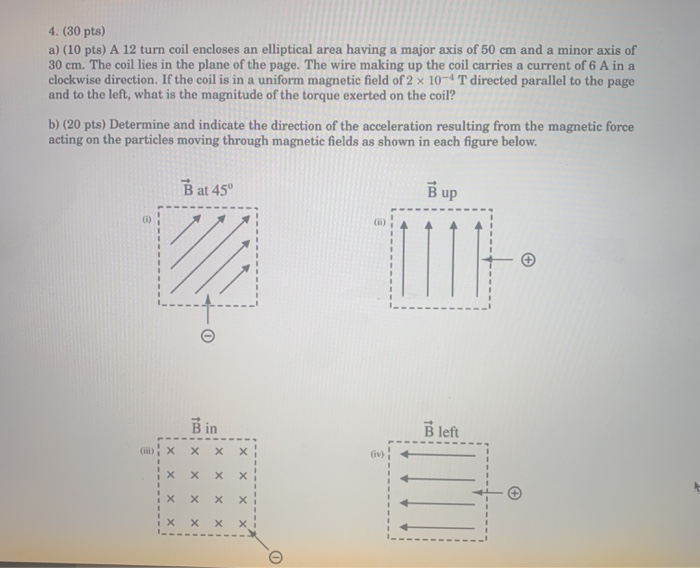Need soon. Please show all work and units. I have some work done but want to receive full points. Thanks! 4. (30 pts) a) (10 pts) A 12 turn coil encloses an elliptical area having a major axis of 50 cm and a minor axis of 30 cm. The coil lies in the plane of the page. The wire making up the coil carries a current of 6 A in a clockwise direction. If the coil is in a uniform...

• ### Please show all work (every bit) and all units. Also please wrote clearly. I need soon....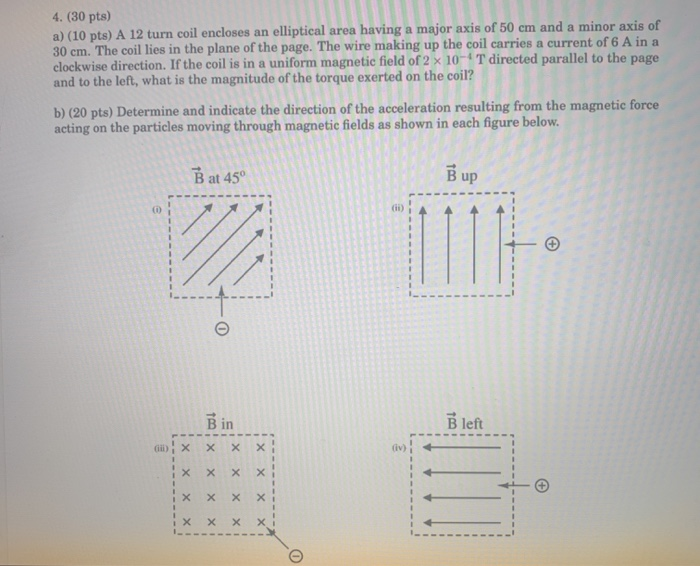Please show all work (every bit) and all units. Also please wrote clearly. I need soon. Thanks. 4. (30 pts) a) (10 pts) A 12 turn coil encloses an elliptical area having a major axis of 50 cm and a minor axis of 30 cm. The coil lies in the plane of the page. The wire making up the coil carries a current of 6 A in a clockwise direction. If the coil is in a uniform magnetic field of...

• ### A 150-turn rectangular coil having dimensions of 10.0 cm by 5.0 cm is placed in a...

A 150-turn rectangular coil having dimensions of 10.0 cm by 5.0 cm is placed in a uniform magnetic field of magnitude of 0.8 T. Assume the max torque exerted on the wire by the magnetic field is 0.12N*m . Find the magnitude of the torque on the coil when the magnetic field makes an angle of 31º with the normal to the plane of the coil .06180457 0.13597005 1.23609138 .61804569

• ### Question 14 3 pts A 250-turn rectangular coil having dimensions of 8.0 cm by 3.0 cm...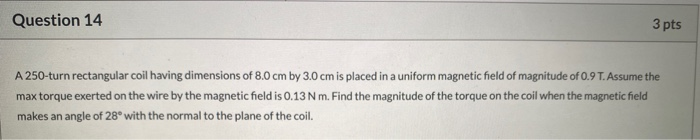Question 14 3 pts A 250-turn rectangular coil having dimensions of 8.0 cm by 3.0 cm is placed in a uniform magnetic field of magnitude of 0.9 T. Assume the max torque exerted on the wire by the magnetic field is 0.13 Nm. Find the magnitude of the torque on the coil when the magnetic field makes an angle of 28° with the normal to the plane of the coil.

• ### Hi there, Can you please check the following? Please check to make sure its correct and...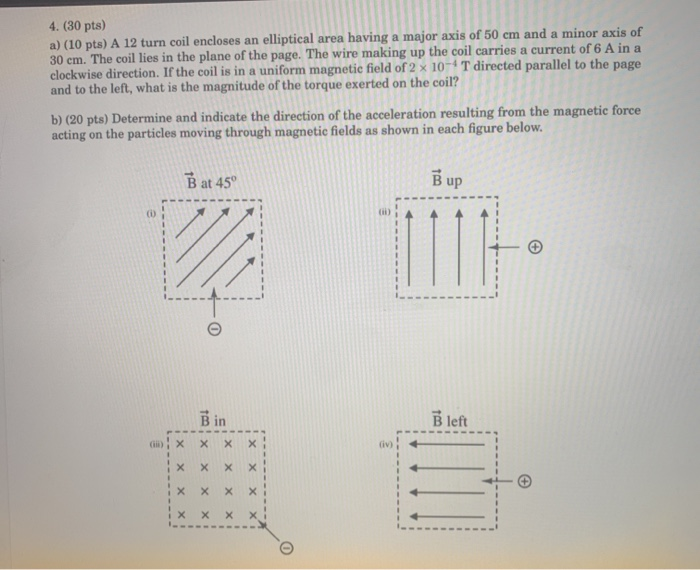Hi there, Can you please check the following? Please check to make sure its correct and all work is shown. I want to receive full pts. if not correct please show all work clearly. thanks 4. (30 pts) a) (10 pts) A 12 turn coil encloses an elliptical area having a major axis of 50 cm and a minor axis of 30 cm. The coil lies in the plane of the page. The wire making up the coil carries a...

• ### A circular coil 14.0 cm in radius and composed of 145 tightly wound turns carries a...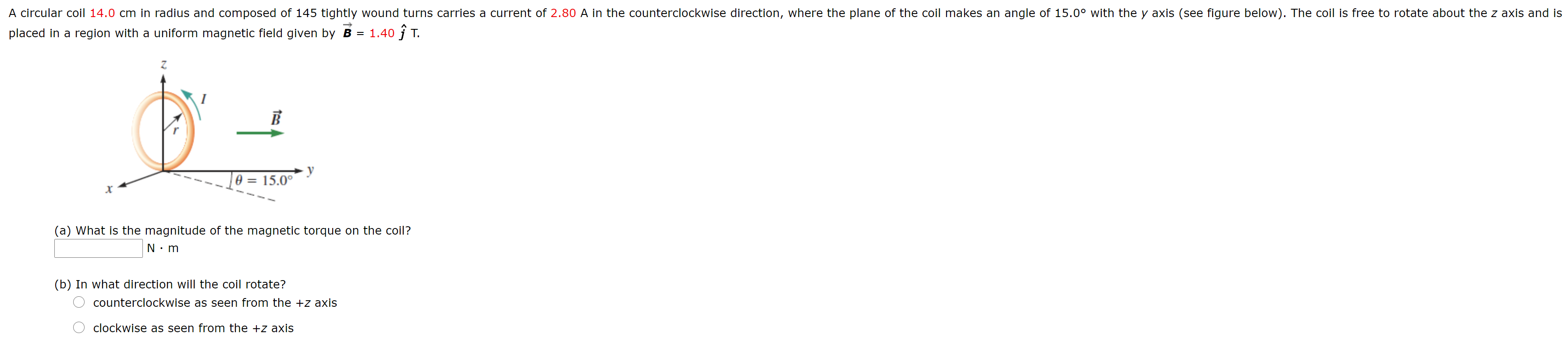A circular coil 14.0 cm in radius and composed of 145 tightly wound turns carries a current of 2.80 A in the counterclockwise direction, where the plane of the coil makes an angle of 15.0° with the y axis (see figure below). The coil is free to rotate about the z axis and is placed in a region with a uniform magnetic field given by B = 1.40 ſ T. B O = 15.0° (a) What is the magnitude of...

Free Homework App